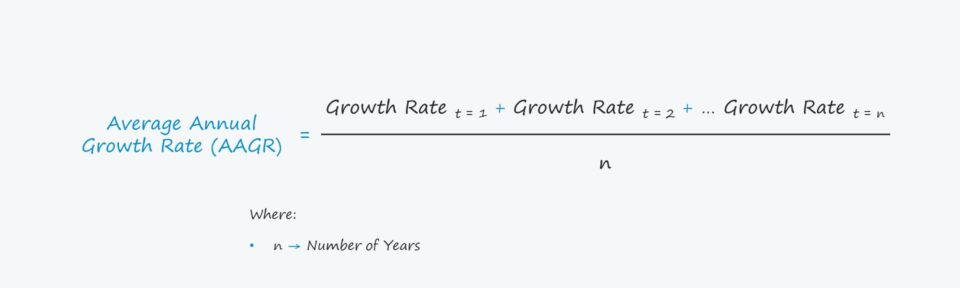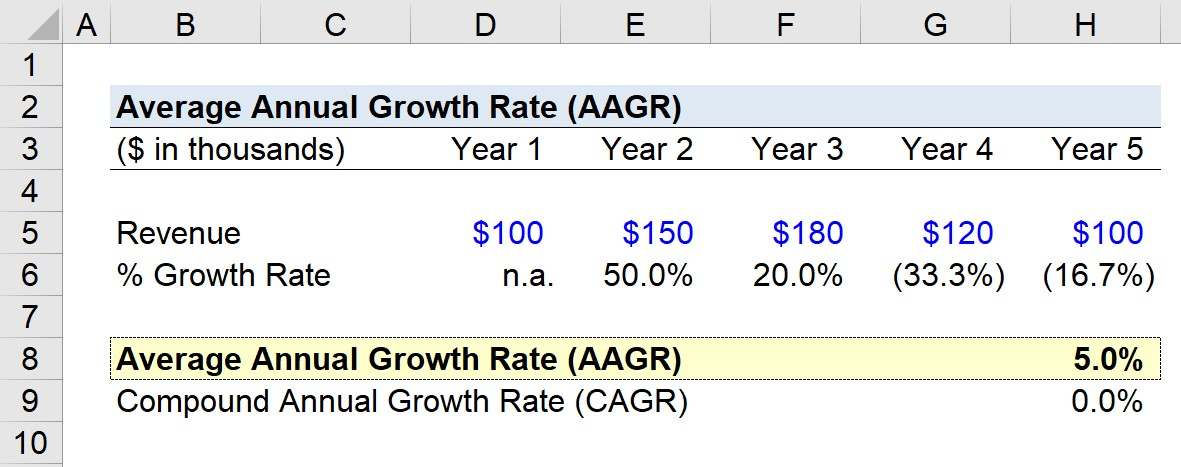# Average Annual Growth Rate (AAGR)

Guide to Understanding Average Annual Growth Rate (AAGR)## How to Calculate the Average Annual Growth Rate (AAGR)

The average annual growth rate refers to the average rate of growth, either positive or negative, related to the value of an investment or portfolio.

In short, the AAGR can be determined by calculating the mean of multiple year-on-year (YoY) growth rates.

When evaluating growth over a multiyear time horizon, the AAGR can be used to assess the average rate of change on an annual basis.

However, while calculating the AAGR, fluctuations that occur in the growth rate from the initial period to the final period are not taken into consideration.

Hence, usage of the AAGR as part of growth analysis is uncommon and is generally avoided.

## AAGR Formula

The formula for calculating the average annual growth rate is as follows.

###### Formula
• Average Annual Growth Rate (AAGR) = (Growth Rate t = 1 + Growth Rate t = 2 + … Growth Rate t = n) / n

Where

• n = Number of Years

## AAGR vs. CAGR

The compound annual growth rate, or “CAGR”, is the annual rate of return required for a metric to grow from its starting balance to its ending balance.

Compared to the compound annual growth rate (CAGR), the average annual growth rate (AAGR) is far less practical since it does not account for the effects of compounding.

In other words, AAGR is a linear measure, whereas CAGR factors in compounding and “smoothens” the growth rate.

For the most part, AAGR is viewed as a simpler, less informative measure because the metric neglects the effects of compounding, a crucial consideration in the context of investing and portfolio management.

Relying on AAGR by itself is not recommended since the volatility risk is ignored.

## Average Annual Growth Rate Calculator – Excel Model Template

We’ll now move to a modeling exercise, which you can access by filling out the form below.Submitting ...

## AAGR Example Calculation

Suppose we’re calculating the average annual growth rate (AAGR) of a company that operates in a highly cyclical industry where demand fluctuates substantially.

The company’s revenue values across a five-year period are as follows:

• Year 1 = \$100k
• Year 2 = \$150k
• Year 3 = \$180k
• Year 4 = \$120k
• Year 5 = \$100k

We’ll calculate the year-over-year (YoY) growth rate for each period by dividing the current period value by the prior period value and then subtracting one.

• Growth Rate Year 1 = n.a.
• Growth Rate Year 2 = 50.0%
• Growth Rate Year 3 = 20.0%
• Growth Rate Year 4 = –33.3%
• Growth Rate Year 5 = –16.7%

If we take the sum of all the growth rates and divide it by the number of years (four years), the average annual growth rate (AAGR) equals 5.0%.

• Average Annual Growth Rate (AAGR) = (50.0% + 20.0% –33.3% –16.7%) / 4 = 5.0%

As a point of comparison, we’ll calculate the CAGR by first taking the ending value and dividing it by the beginning value.

Next, we’ll raise the resulting figure to the power of one divided by the number of years and conclude by subtracting one.

• CAGR = (\$100k / \$100k)^(1/4) – 1 = 0%

The CAGR comes out to 0%, showing why reliance on AAGR alone (or without the proper context) can easily be misleading.

Based on our assumptions, it is clear that our company’s revenue is volatile (and thus risky), yet the 5.0% AAGR does not necessarily reflect that.Step-by-Step Online Course

### Everything You Need To Master Financial Modeling

Enroll in The Premium Package: Learn Financial Statement Modeling, DCF, M&A, LBO and Comps. The same training program used at top investment banks.